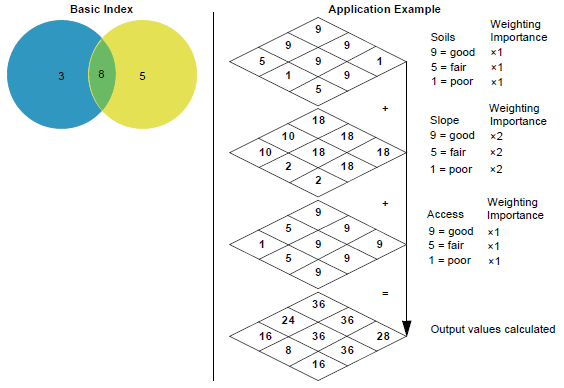# Indexing

## Producer Field Guide

HGD_Product
Producer Field Guide
HGD_Portfolio_Suite
Producer

Thematic layers can be indexed (added) to create a composite layer. The output layer contains the sums of the input layer values. For example, the intersection of class 3 in one layer and class 5 in another would result in class 8 in the output layer, as shown in the figure below.

IndexingThe application example in the figure above shows the result of indexing. In this example, you want to develop a new subdivision, and the most likely sites are where there is the best combination (highest value) of good soils, good slope, and good access. Because good slope is a more critical factor to you than good soils or good access, a weighting factor is applied to the slope layer. A weighting factor has the effect of multiplying all input values by some constant. In this example, slope is given a weight of 2.

Use the Index (GIS Analysis) function in the IMAGINE Workspace or Spatial Modeler to index layers.